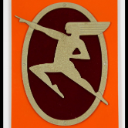# Help with dates in arcade?

910
3
12-17-2019 09:39 AMOccasional Contributor

Hi Friends,

I have a little python script that calculates  whether this week is an even week or an odd week.
I need this in arcade to populate in an AGOL app but I am below beginner level with arcade and finding it difficult to find samples to work with.

Does anybody have the ability to help me put together a small arcade  expression that does what my python does or else point me to where I can find some samples?  There is a world of python samples out there, but arcade is harder to find.

from datetime import *

#make sure to always start on a monday
startDate = date(2019, 12, 2)

todayDate = date.today()

# count the days between the dates
delta = todayDate - startDate

#count the number of weeks since start date
weekNum = delta.days / 7

#determine if this week is even or odd
# Python program to check if the input number is odd or even.
# A number is even if division by 2 gives a remainder of 0.
# If the remainder is 1, it is an odd number.
num = int(weekNum)
if (num % 2) == 0:

print("{0} is Even".format(num))
print "This week is paper recycling"

else:

print("{0} is Odd".format(num))
print "This week is container recycling"

Tags (3)
1 Solution

Accepted SolutionsbyMVP Honored Contributor

Give this a try

``````var startDate = Date(2019, 11, 2); //Months are 0-11
var todayDate = Now();

var delta = Abs(DateDiff(startDate, todayDate, "days"));
var weekNum = Floor(delta/7);
if ((weekNum % 2) == 0){
return "even" + ", " + delta + ", " + weekNum;
} else {
return "odd"+ ", " + delta + ", " + weekNum;
}‍‍‍‍‍‍‍‍‍‍``````
3 RepliesbyMVP Honored Contributor

Give this a try

``````var startDate = Date(2019, 11, 2); //Months are 0-11
var todayDate = Now();

var delta = Abs(DateDiff(startDate, todayDate, "days"));
var weekNum = Floor(delta/7);
if ((weekNum % 2) == 0){
return "even" + ", " + delta + ", " + weekNum;
} else {
return "odd"+ ", " + delta + ", " + weekNum;
}‍‍‍‍‍‍‍‍‍‍``````Occasional Contributor

Ken Buja, you are the best, most capable Bro on GeoNet today.

I was all Ric Flair over here when I saw your response.

3 gold stars!Occasional Contributor

Ken Buja, you are the best, most capable Bro on GeoNet today.

I was all Ric Flair over here when I saw your response.

3 gold stars!